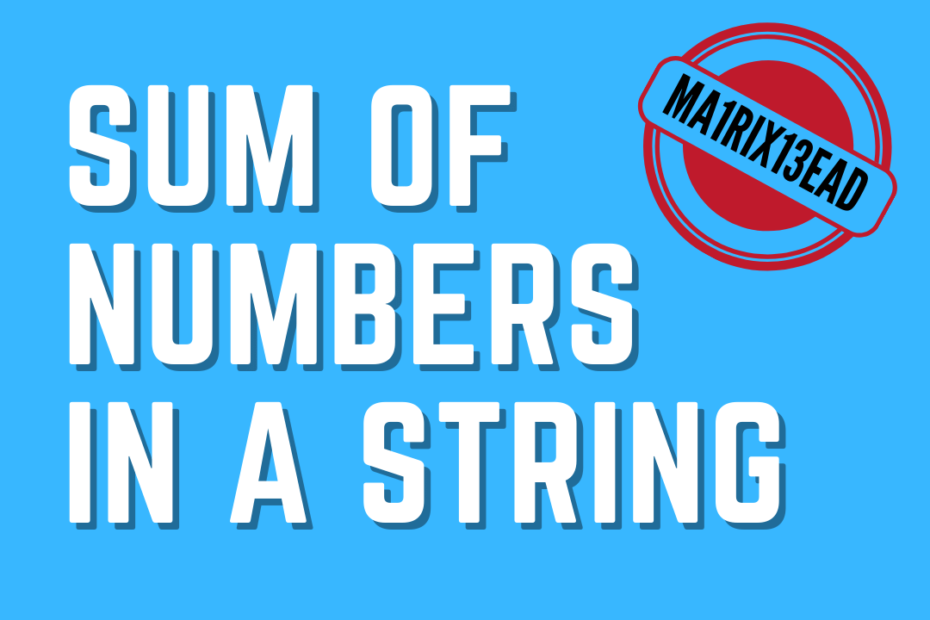# Sum of Numbers in a String

• DSA

Today, I found a problem where we need to find the sum of numbers in a string, the string will be a mix of alphabets, numbers, and special characters, and usually, this can be solved by iterating through each character and adding all the numbers.

## Example

``````Input: Ma1rix13ead
Output: 14  [1+13]

Input: Abhi12xyz3pq4
Output: 19  [12+3+4]``````

## Solution Approach

Given a string made up of numbers, alphabets, and other special characters. But, we need to collect all the numbers present and print their sum. We can start iterating through each character in the string and add them if it’s a number and this can be done easily as shown below

``````isdigit(character)
sum+=int(character)``````

But, What if the string contains continuous numbers?

In `AB12C4` we can’t just add 1+2+4

And to do that, we need to check whether the next character is also a number and then proceed to addition.

## Pseudocode

1. Iterate through all characters in the String
2. If it’s a number save it
3. If the next character is also a number save it
4. If the next character is not a number
5. Combine all the saved numbers and Add

## Code

#### Sum of Numbers in a String C++

``````#include <iostream>
using namespace std;

// Sum of Numbers in a String
int NumSum(string str)
{
string temp = "";
int sum = 0;
//iterate through the whole string
for (char ch : str)
{
//keeps collecting continous numbers
if (isdigit(ch))
temp += ch;
else
{
//atoi convers a string of numbers to integer
// 20 is converted to 20
sum += atoi(temp.c_str());
temp = "";
}
}
return sum + atoi(temp.c_str());
}

int main()
{
``100``